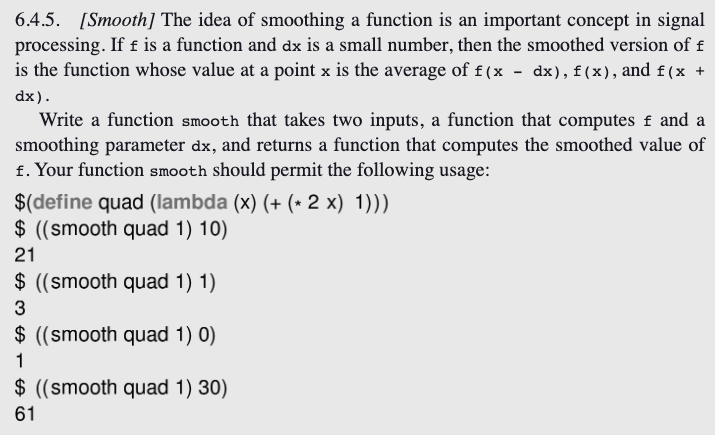Rating : ⭐⭐⭐⭐⭐
Price : \$10.99
Language:EN
Pages: 3
Words: 327

# Smooth Function with Smoothing Homework Answers Needed

6.4.5. [Smooth] The idea of smoothing a function is an important concept in signal processing. If f is a function and dx is a small number, then the smoothed version of f is the function whose value at a point x is the average of f(x - dx), f(x), and f (x + dx).

Write a function smooth that takes two inputs, a function that computes f and a

21

61# Step By Step Answers with Explanation

4. Return the inner function as the result of the `smooth` function.

Here's the code in Scheme:

(+ (* 2 x) 1))

(newline)

(display ((smoothed-quad 0)))  ; Output: 1

1. We define the `smooth` function, which takes `f` and `dx` as arguments.

2. Inside the `smooth` function, we define an inner function that takes a value `x`.

How It Works